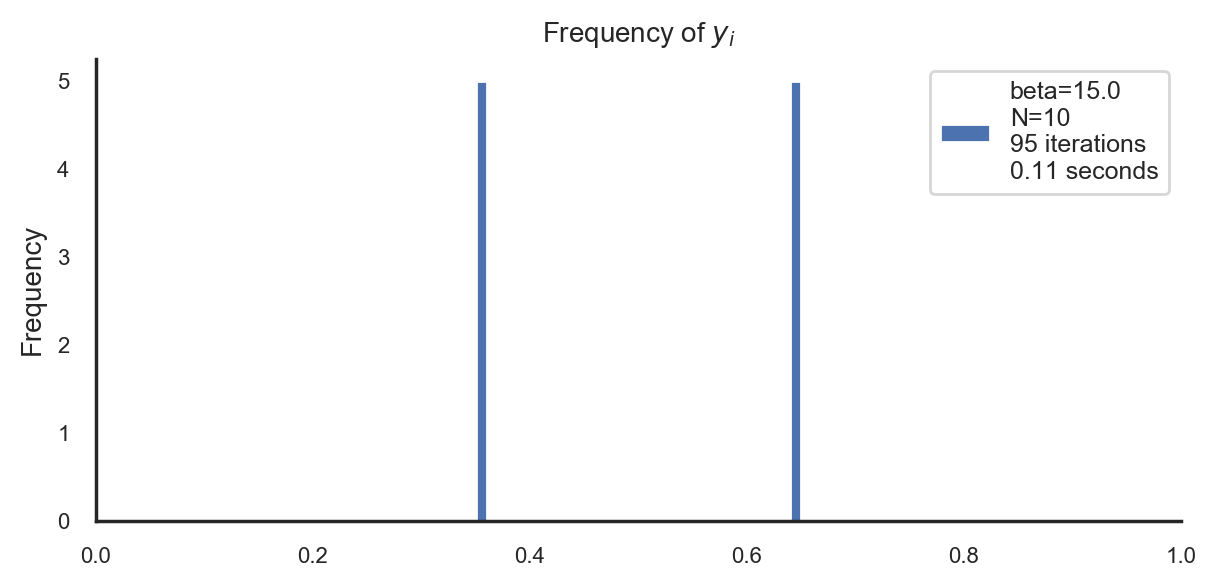This notebook should be viewed on nbviewer.jupyter.org to render properly.
In :
from IPython.core.display import HTML

Out:
In :
import urllib
response = urllib.request.urlopen("https://gist.githubusercontent.com/sgttwld/9deff60facb16918e54410cca3d65648/raw")
HTML("<style type='text/css'>"+css+"</style>")

Out:
In :
%config InlineBackend.figure_format = 'retina'

In :
# imports
import numpy as np
import matplotlib.pyplot as plt
import seaborn as sns
import pandas as pd
import tensorflow as tf
from RD_BA import BA
from RD_GD import GD
from RD_MA import MA, MA_iter

In :
# plot settings
sns.reset_defaults()
sns.set(
rc={
'figure.figsize': (7,3),
'axes.titlesize': 10,
'axes.labelsize': 10,
'legend.fontsize': 9,
'xtick.labelsize': 8,
'ytick.labelsize': 8,
'axes.spines.top': False,
'axes.spines.right': False,
},
style="white"
)

In :
def get_source(num):
return tf.constant( np.random.beta(a=2,b=2,size=(num)) )

In :
def legend_string(r):
return ['beta={}\nN={}\n{} iterations\n{:.2f} seconds'.format(
r['beta'],len(r['xhat']),r['episodes'],r['elapsed'])]

In :
# parameters
beta = 15.0
num = 5000


# Rate-distortion: Blahut-Arimoto vs. gradient descent vs. mapping approach¶

Consider a continuous source $X$, for example distributed according to a beta distribution:

In :
# source
X = get_source(num)
ax = pd.DataFrame(X).plot.hist(bins=20,title='Source frequency')
ax.legend([r'X ~ Beta(2,2)'])
plt.show()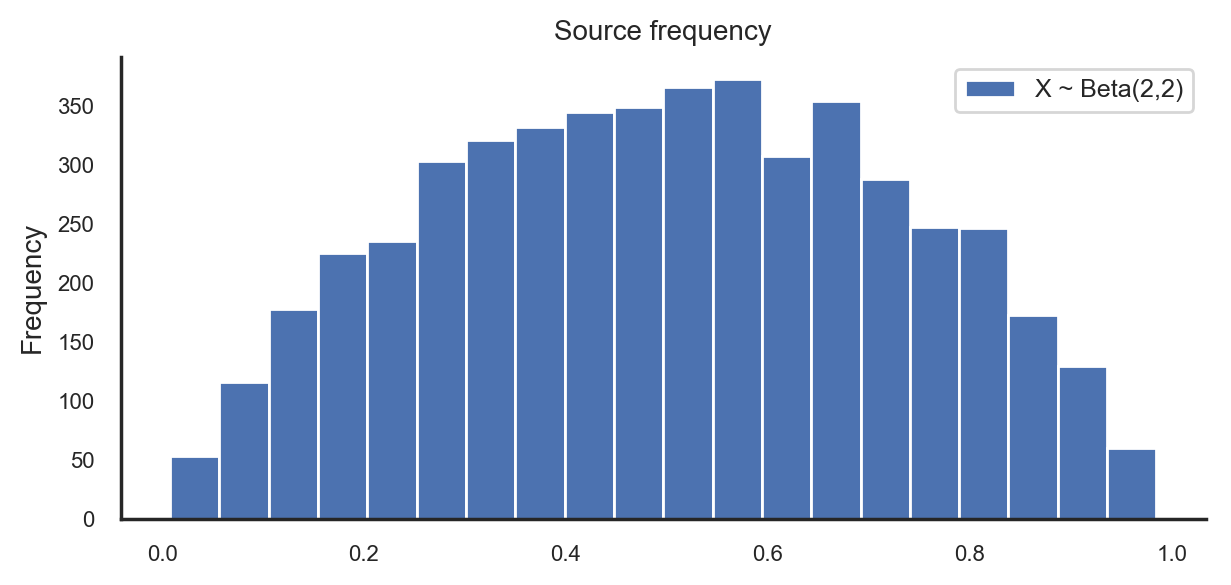Below we compare three algorithms that are designed to find the minimum of the rate-distortion Lagrangian (a.k.a. free energy) $$F_{RD}(q(\hat X|X)) := \mathbb E[d(X,\hat X)] + \tfrac{1}{\beta} \, I(X;\hat X)$$ with respect to conditional distributions $q(\hat X|X)$, where $d$ denotes a distortion function (e.g. the squared distance $d(s,t) = (s-t)^2$, which is chosen in the simulations below), and $$I(X;\hat X) = \mathbb E_X [D_{KL}(q(\hat X|X)\| \mathbb E_X[q(\hat X|X)])]$$ is the mutual information between the source $X$ and the reconstruction $\hat X$. All three algorithms make use of the fact that the rate-distortion Lagrangian $F_{RD}$ can be written as the optimum $$F_{RD}(q(\hat X|X)) = \min_{q(\hat X)} F^{aux}_{RD}(q(\hat X|X), q(\hat X))$$ where the auxiliary Lagrangian is defined as $$F^{aux}_{RD}(q(\hat X|X), q(\hat X)):= \mathbb E[d(X,\hat X)] + \tfrac{1}{\beta} \, \mathbb E_X [D_{KL}(q(\hat X|X)\| q(\hat X))] ,$$ since the optimal reconstruction distribution $q^\ast(\hat X)$ is simply given by the marginal $\mathbb E_X[q(\hat X|X)],$ and by construction we have $F_{RD}(\, \cdot \, ) = F^{aux}_{RD}(\, \cdot \, ,\mathbb E_X[q(\hat X|X)])$.

## 1. Blahut-Arimoto (RD_BA.py)¶

When the Blahut-Arimoto algorithm is applied to rate-distortion, the auxiliary Lagrangian $F^{aux}_{RD}$ is optimized alternatingly with respect to $q(\hat X|X)$ and $q(\hat X)$ by iterating the closed-form solutions $$q^\ast(\hat X|X=x) = \frac{1}{Z(x)} q(\hat X) \, e^{-\beta d(x,\hat X)} \ , \ \ q^\ast(\hat X) = \mathbb E_X[q(\hat X|X)]$$ where $Z(x) := \mathbb E_{q(\hat X)}[e^{-\beta d(x,\hat X)}]$.

This is often done by discretizing the range of $X$ (here the interval $[0,1]$) into $N$ fixed parts, so that $\hat X$ has finite range (one value for each interval) and its probability distribution can be represented by an $N$-dimensional probability vector. The Blahut-Arimoto algorithm then iterates between (i) calculating the Boltzmann distribution $q^\ast(\hat X|X=x)$ for each sample $x$, and (ii) averaging the results over all samples in the dataset to obtain $q(\hat X)$.

In :
# Blahut-Arimoto
r = BA(X,beta,N=40)
ax = pd.DataFrame(r['q']).plot.bar(title=r'Distribution of reconstruction $\hat{X}$',rot=0)
ax.legend(legend_string(r))
xticks = np.arange(0,len(r['q']),8)
plt.xticks(xticks,np.round(r['xhat'],2)[xticks])
plt.show()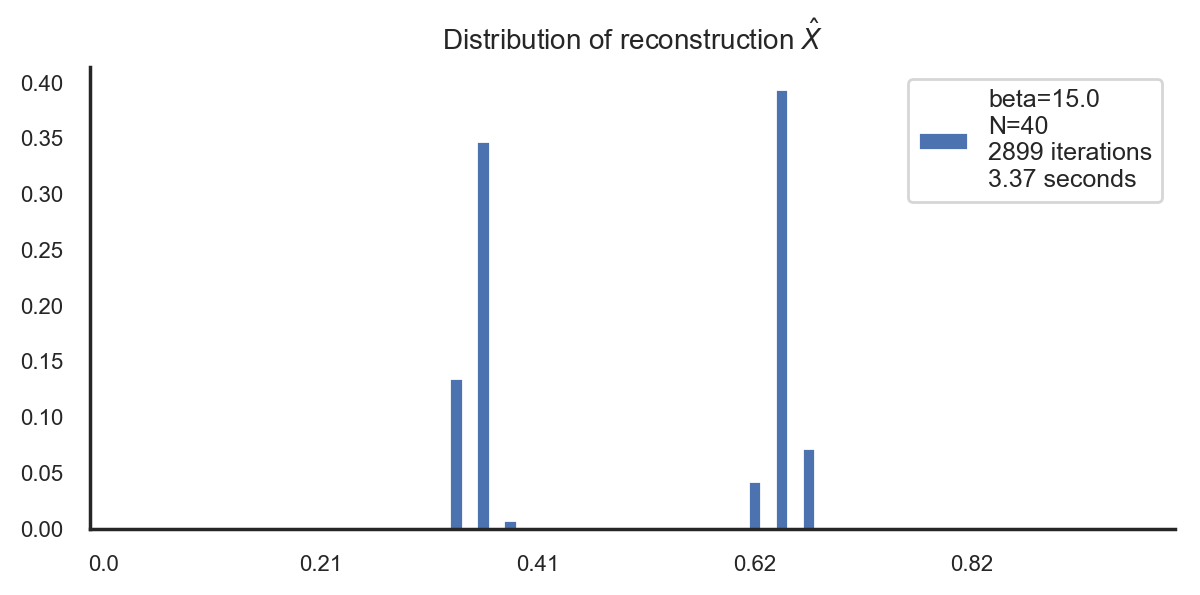Here, we use that evaluating the auxiliary free energy $F^{aux}_{RD}$ at the Boltzmann distribution $q^\ast(\hat X|X)$ results in $$F_{GD}(q(\hat X)) := F^{aux}_{RD}(q^\ast(\hat{X}|X), q(\hat{X})) = -\frac{1}{\beta}\mathbb{E}_X \Big[ \log \mathbb E_{q(\hat{X})} [e^{-\beta d(X,\hat{X})}] \Big].$$ which can be optimized with respect to $q(\hat{X})$ by using gradient descent. In particular, exactly as in the Blahut-Arimoto algorithm, we discretize the range of $X$ into $N$ fixed chunks, which transforms the infinite-dimensional optimization problem over distributions $q(\hat{X})$ into an $N$-dimensional optimization problem over probability vectors.

In :
r = GD(X,beta,N=40)
ax = pd.DataFrame(r['q']).plot.bar(title=r'Distribution of reconstruction $\hat{X}$',rot=0)
ax.legend(legend_string(r))
xticks = np.arange(0,len(r['q']),8)
plt.xticks(xticks,np.round(r['xhat'],2)[xticks])
plt.show()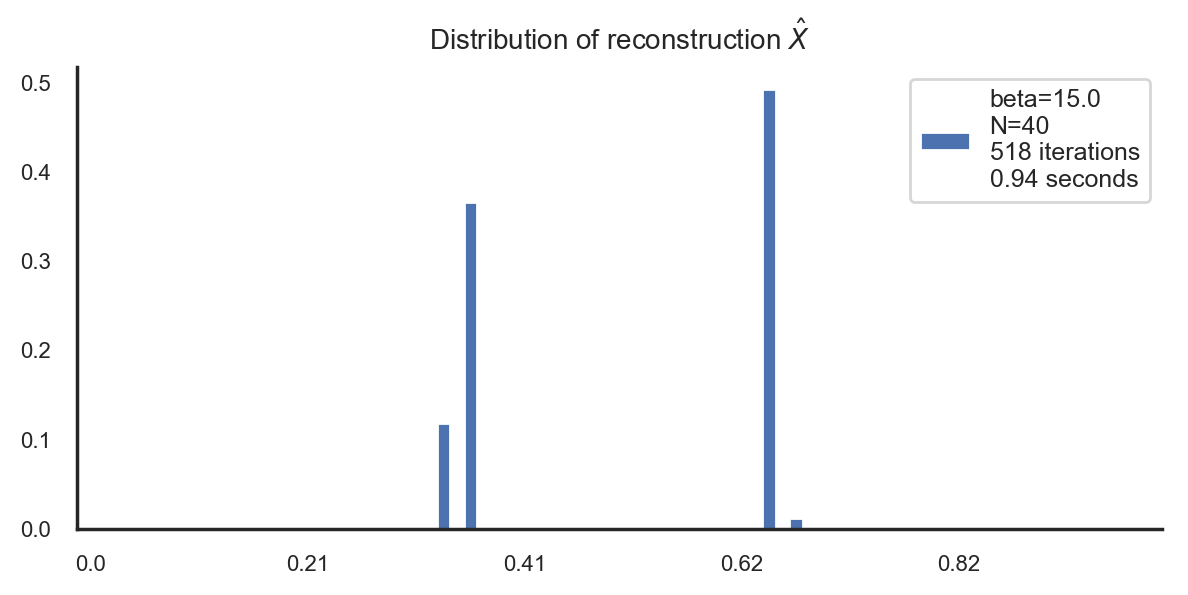Note that the above results imply that for our choice of $\beta=15$ the optimal reconstruction has support on only two elements (which are located such that the average distortion is minimized). By increasing $\beta$, there will be several phase transitions where more and more reconstruction points are added. However, due to the fixed discretization of the range of $X$, the exact support and shape of $q(\hat X)$ can only be approximated. This issue is resolved by the following approach.

## 3. Mapping approach (RD_MA.py)¶

The mapping approach to rate-distortion makes use of the Borel isomorphism theorem, which allows to replace the expectation with respect to $q(\hat{X})$ by integration over $u\in [0,1]$ (with respect to the Lebesgue measure) and where the value of $\hat X$ is replaced by the value $y(u)$ of some mapping $y:[0,1]\to \mathrm{ran}(X)$, i.e. $$\mathbb E_{q(\hat X)}[f(\hat X)] = \int_0^1 f(y(u)) du$$ for any measurable function $f$. Applied to the minimization of the Lagrangian $F_{GD}$ above, this allows to replace the optimization of $F_{GD}$ with respect to $q(\hat X)$ by the optimization of $$F_{M}(y) := -\frac{1}{\beta}\mathbb{E}_X \Big[ \log \int_0^1 e^{-\beta d(X,y(u))} du \Big]$$ with respect to the mappings $y:[0,1]\to \mathrm{ran}(X)$.

Instead of discretizing the range of $X$, we can now discretize the domain of $y$, so that the optimization is over the values $y_i := y(b_i)$ where $(b_i)_{i=1}^N$ are the fixed representatives for each chunk of the discretization of $[0,1]$. Note that the difference to the other approaches boils down to how the expectation with respect to $q(\hat X)$ is calculated from an $N$-dimensional vector, $$\underbrace{\sum_{i=1}^N q_i e^{-\beta d(X,\hat x_i)}}_{\text{1. and 2. above}} \ \ vs. \ \ \underbrace{\frac{1}{N}\sum_{i=1}^N e^{-\beta d(X,y_i)}}_{\text{mapping approach}}$$ where $\{\hat x_i\}_{i=1}^N$ is the fixed finite range of $\hat X$ and $q_i:=q(\hat x_i)$ are the optimization variables in the above approaches, whereas $y_i = y(b_i)$ are the optimization variables in the mapping approach. In particular, here the possible values of the reconstruction $\hat X$ can perfectly adapt to the source $X$, whereas in the other approaches the possible values are fixed by the discretization. We have implemented two different variants of the mapping approach:

Direct optimization. Here we optimize the discretized version of $F_M$, $$F^{disc}_M(y) := - \frac{1}{\beta} \mathbb E_X\Big[ \log \sum_{i=1}^N e^{-\beta d(X,y_i)} \Big] ,$$ directly with respect to $y=(y_i)_{i=1}^N$ using gradient descent.

In :
r = MA(X,beta,N=10)
ax = pd.DataFrame(r['xhat']).plot.hist(bins=100,range=(0,1),title=r'Frequency of $y_i$')
ax.legend(legend_string(r))
plt.xlim([0,1])
plt.show()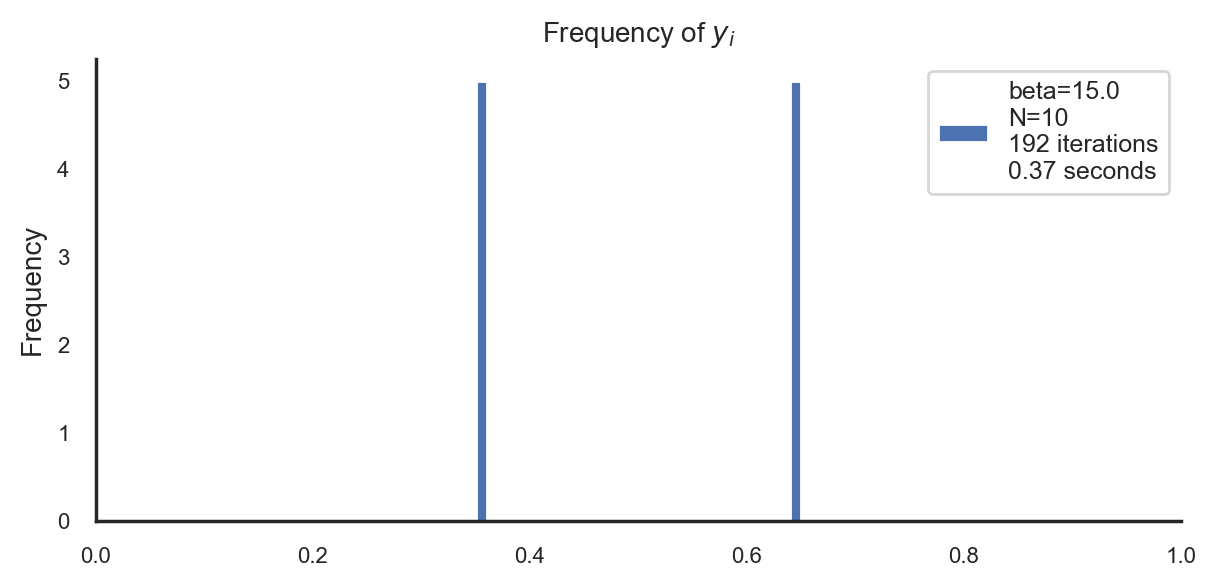Iterative optimization. This variant is similar to the Blahut-Arimoto algorithm above, in that it makes use of the fact that the discretized version of $F_M(y)$ can be written as $$F^{disc}_M(y) = \min_{q(I|X)} F^{aux}_M(y,q(I|X))$$ with the auxiliary Lagrangian (see e.g. Gottwald, Braun 2020) $$F^{aux}_M(y,q(I|x)) := \mathbb E_{X} \Big[ \mathbb E_{q(I|X)}[d(X,y_I)] + \tfrac{1}{\beta} D_{KL}(q(I|X)\|\tfrac{1}{N})\Big].$$ Minimizing $F'_{aux}$ separately in each argument yields the closed-form solutions $$q^\ast(I|X=x) = \frac{e^{-\beta d(x,y_i)}}{\sum_j e^{-\beta d(x,y_j)}} \ , \ \ y^\ast_i = E[X|I{=}i] = \frac{\mathbb E_X[X q(i|X)]}{E_X[q(i|X)]}$$ where the solution for $y^\ast$ is only valid for Bregman distortion measures (see e.g. Banerjee 2005). Iterating these equation yields an algorithm similar to the Blahut-Arimoto algorithm, which will have performance characteristics that are different from the direct optimization above.

In :
# Mapping approach (iterative algorithm)
r = MA_iter(X,beta,N=10)
ax = pd.DataFrame(r['xhat']).plot.hist(bins=100,range=(0,1),title=r'Frequency of $y_i$')
ax.legend(legend_string(r))
plt.xlim([0,1])
plt.show()